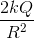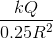# MCAT Physical : Electric Fields

## Example Questions

### Example Question #6 : Electrostatics And Electrical Fields

Batteries and AC current are often used to charge a capacitor. A common example of capacitor use is in computer hard drives, where capacitors are charged in a specific pattern to code information. A simplified circuit with capacitors can be seen below. The capacitance of C1 is 0.5 μF and the capacitances of C2 and C3 are 1 μF each. A 10 V battery with an internal resistance of 1 Ω supplies the circuit.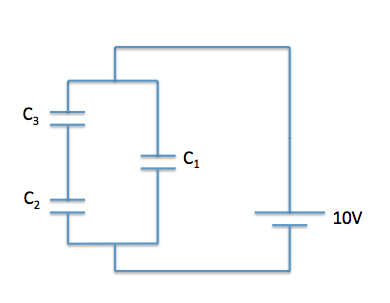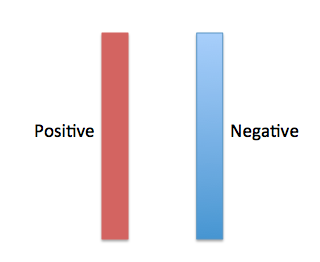Using the plate diagram above, what direction do the electric field lines point?

To the left

Into the page

Out of the page

To the right

To the right

Explanation:

As with a point charge, remember that electric field lines point from areas of high potential to areas of low potential. Areas of high potential have positive (+) charge and areas of low potential have negative (-) charge, thus the electric field lines point to the right.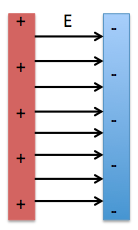### Example Question #7 : Electrostatics And Electrical Fields

Which of the following equations best relates capacitance, charge, and distance between parallel plates as they pertain to the electric field,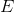?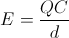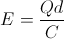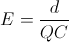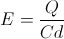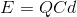Explanation:

This question forces us to combine two equations.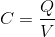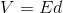Using substitution, we can solve for the electric field with the variables given in the question stem.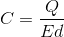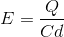### Example Question #8 : Electrostatics And Electrical Fields

Batteries and AC current are often used to charge a capacitor. A common example of capacitor use is in computer hard drives, where capacitors are charged in a specific pattern to code information. A simplified circuit with capacitors can be seen below. The capacitance of C1 is 0.5 μF and the capacitances of C2 and C3 are 1 μF each. A 10 V battery with an internal resistance of 1 Ω supplies the circuit.Using the plate diagram above, in what direction do the electric force lines point?

Into the page

To the left

Out of the page

To the right

To the right

Explanation:

As with a point charge, remember that electric force lines point away from positive charge and towards negative charge. As can be seen in the image, electric force lines point to the right. As an aside, electric field lines point in the same direction as electric force lines, so if you know the direction of one, you also know the direction of the other.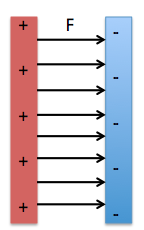### Example Question #9 : Electrostatics And Electrical Fields

Consider a spherical shell of radiushas a charge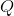.

What is the electric field at a distance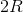from the surface of the shell?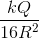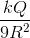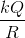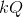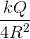Explanation:

According to the shell theorem, a charged spherical shell can be treated as a point charge at the center of the spherical shell. The equation for the electric field of a point charge is: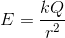In this case,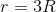since we are at a distance offrom the surface of the shell and therefore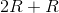away from the shell's center. Plug into the equation for electric field.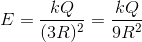### Example Question #10 : Electrostatics And Electrical Fields

Consider a spherical shell of radiushas a charge.

What is the electric field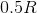away from the center of the shell?